# NCERT Solutions for Class 9 Maths Chapter 7 Triangles

NCERT Solutions for Class 9 Maths Chapter 7 Triangles

## NCERT Solutions for Class 9 Maths Chapter 7 Triangles Ex 7.1

Ex 7.1 Class 9 Maths Question 1.
In quadrilateral ACBD, AC = AD and AB bisects ∠ A (see figure). Show that ∆ABC ≅ ∆ABD. What can you say about BC and BD?Solution:
In quadrilateral ACBD, we have AC = AD and AB being the bisector of ∠A.
Now, In ∆ABC and ∆ABD,
∠ CAB = ∠ DAB ( AB bisects ∠ CAB)
and AB = AB (Common)
∴ ∆ ABC ≅ ∆ABD (By SAS congruence axiom)
∴ BC = BD (By CPCT)

Ex 7.1 Class 9 Maths Question 2.
ABCD is a quadrilateral in which AD = BC and ∠ DAB = ∠ CBA (see figure). Prove that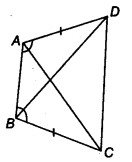(i) ∆ABD ≅ ∆BAC
(ii) BD = AC
(iii) ∠ABD = ∠ BAC
Solution:
In quadrilateral ACBD, we have AD = BC and ∠ DAB = ∠ CBA

(i) In ∆ ABC and ∆ BAC,
∠DAB = ∠CBA (Given)
AB = AB (Common)
∴ ∆ ABD ≅ ∆BAC (By SAS congruence)

(ii) Since ∆ABD ≅ ∆BAC
⇒ BD = AC [By C.P.C.T.]

(iii) Since ∆ABD ≅ ∆BAC
⇒ ∠ABD = ∠BAC [By C.P.C.T.]

Ex 7.1 Class 9 Maths Question 3.
AD and BC are equal perpendiculars to a line segment AB (see figure). Show that CD bisects AB.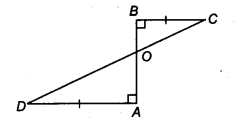Solution:
In ∆BOC and ∆AOD, we have
∠BOC = ∠AOD
∠BOC = ∠AOD [Vertically opposite angles]
∴ ∆OBC ≅ ∆OAD [By AAS congruency]
⇒ OB = OA [By C.P.C.T.]
i.e., O is the mid-point of AB.
Thus, CD bisects AB.

Ex 7.1 Class 9 Maths Question 4.
l and m are two parallel lines intersected by another pair of parallel lines p and q (see figure). Show that ∆ABC = ∆CDA.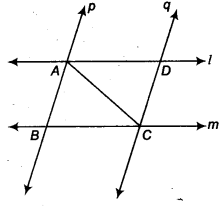Solution:
∵ p || q and AC is a transversal,
∴ ∠BAC = ∠DCA …(1) [Alternate interior angles]
Also l || m and AC is a transversal,
∴ ∠BCA = ∠DAC …(2)
[Alternate interior angles]
Now, in ∆ABC and ∆CDA, we have
∠BAC = ∠DCA [From (1)]
CA = AC [Common]
∠BCA = ∠DAC [From (2)]
∴ ∆ABC ≅ ∆CDA [By ASA congruency]

Ex 7.1 Class 9 Maths Question 5.
Line l is the bisector of an ∠ A and ∠ B is any point on l. BP and BQ are perpendiculars from B to the arms of LA (see figure). Show that
(i) ∆APB ≅ ∆AQB
(ii) BP = BQ or B is equidistant from the arms ot ∠A.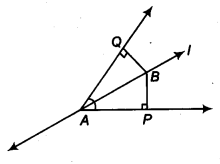Solution:
We have, l is the bisector of ∠QAP.
∴ ∠QAB = ∠PAB
∠Q = ∠P [Each 90°]
∠ABQ = ∠ABP
[By angle sum property of A]
Now, in ∆APB and ∆AQB, we have
∠ABP = ∠ABQ [Proved above]
AB = BA [Common]
∠PAB = ∠QAB [Given]
∴ ∆APB ≅ ∆AQB [By ASA congruency]
Since ∆APB ≅ ∆AQB
⇒ BP = BQ [By C.P.C.T.]
i. e., [Perpendicular distance of B from AP]
= [Perpendicular distance of B from AQ]
Thus, the point B is equidistant from the arms of ∠A.

Ex 7.1 Class 9 Maths Question 6.
In figure, AC = AE, AB = AD and ∠BAD = ∠EAC. Show that BC = DE.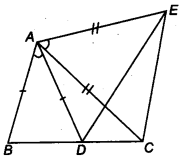Solution:
Adding ∠DAC on both sides, we have
∠BAD + ∠DAC = ∠EAC + ∠DAC
⇒ ∠BAC = ∠DAE
Now, in ∆ABC and ∆ADE. we have
∠BAC = ∠DAE [Proved above]
AC = AE [Given]
∴ ∆ABC ≅ ∆ADE [By SAS congruency]
⇒ BC = DE [By C.P.C.T.]

Ex 7.1 Class 9 Maths Question 7.
AS is a line segment and P is its mid-point. D and E are points on the same side of AB such that ∠ BAD = ∠ ABE and ∠ EPA = ∠ DPB. (see figure). Show that
(i) ∆DAP ≅ ∆EBP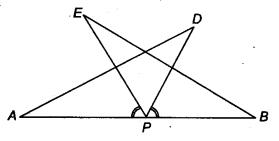Solution:
We have, P is the mid-point of AB.
∴ AP = BP
∠EPA = ∠DPB [Given]
Adding ∠EPD on both sides, we get
∠EPA + ∠EPD = ∠DPB + ∠EPD
⇒ ∠APD = ∠BPE

(i) Now, in ∆DAP and ∆EBP, we have
AP = BP [Proved above]
∠DPA = ∠EPB [Proved above]
∴ ∆DAP ≅ ∆EBP [By ASA congruency]

(ii) Since, ∆ DAP ≅ ∆ EBP
⇒ AD = BE [By C.P.C.T.]

Ex 7.1 Class 9 Maths Question 8.
In right triangle ABC, right angled at C, M is the mid-point of hypotenuse AB. C is joined to M and produced to a point D such that DM = CM. Point D is joined to point B (see figure). Show that
(i) ∆AMC ≅ ∆BMD
(ii) ∠DBC is a right angle
(iii) ∆DBC ≅ ∆ACB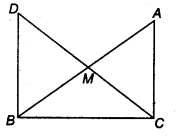(iv) CM = 1/2 AB
Solution:
Since M is the mid – point of AB.
∴ BM = AM

(i) In ∆AMC and ∆BMD, we have
CM = DM [Given]
∠AMC = ∠BMD [Vertically opposite angles]
AM = BM [Proved above]
∴ ∆AMC ≅ ∆BMD [By SAS congruency]

(ii) Since ∆AMC ≅ ∆BMD
⇒ ∠MAC = ∠MBD [By C.P.C.T.]
But they form a pair of alternate interior angles.
∴ AC || DB
Now, BC is a transversal which intersects parallel lines AC and DB,
∴ ∠BCA + ∠DBC = 180° [Co-interior angles]
But ∠BCA = 90° [∆ABC is right angled at C]
∴ 90° + ∠DBC = 180°
⇒ ∠DBC = 90°

(iii) Again, ∆AMC ≅ ∆BMD [Proved above]
∴ AC = BD [By C.P.C.T.]
Now, in ∆DBC and ∆ACB, we have
BD = CA [Proved above]
∠DBC = ∠ACB [Each 90°]
BC = CB [Common]
∴ ∆DBC ≅ ∆ACB [By SAS congruency]

(iv) As ∆DBC ≅ ∆ACB
DC = AB [By C.P.C.T.]
But DM = CM [Given]
∴ CM = 1/2DC = 12AB
⇒ CM = 1/2AB

### NCERT Solutions for Class 9 Maths Chapter 7 Triangles Ex 7.2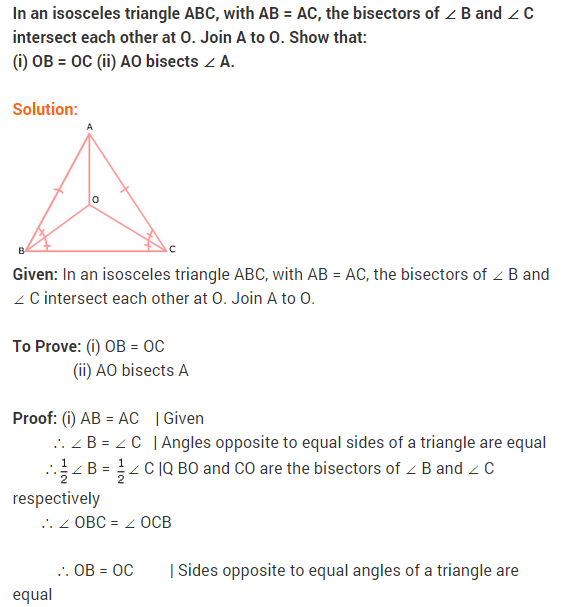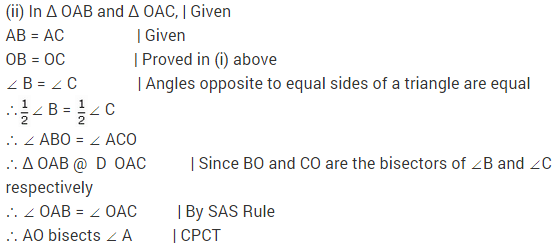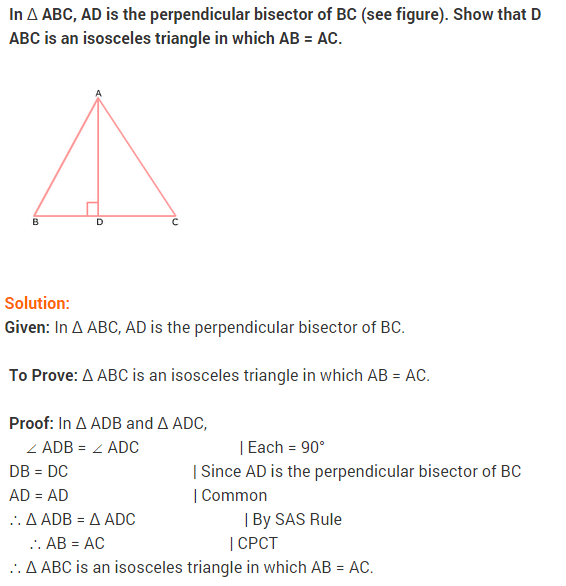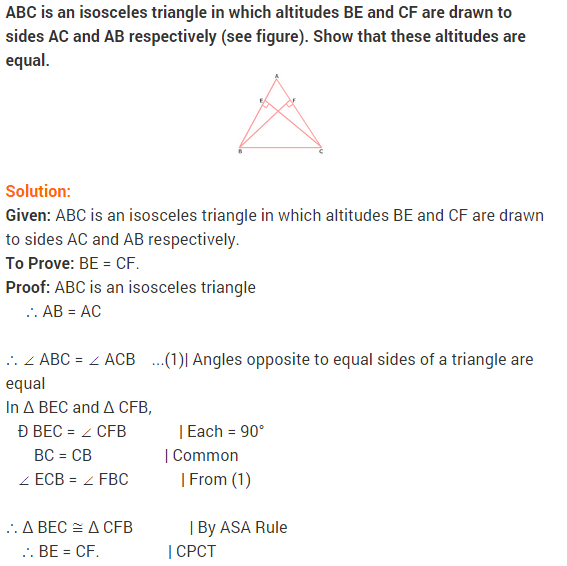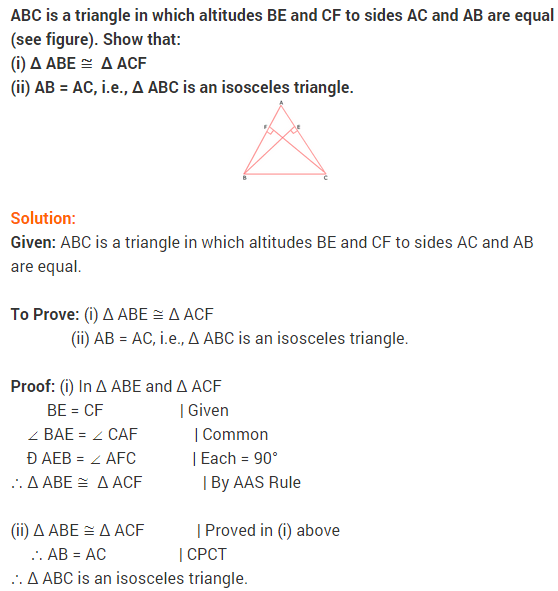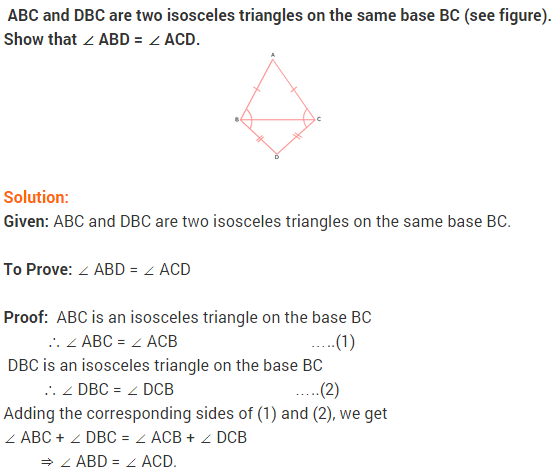### NCERT Solutions for Class 9 Maths Chapter 7 Triangles Ex 7.3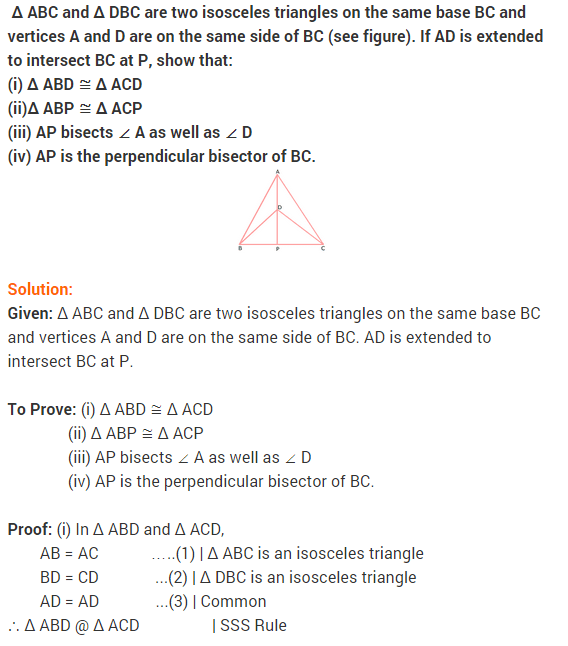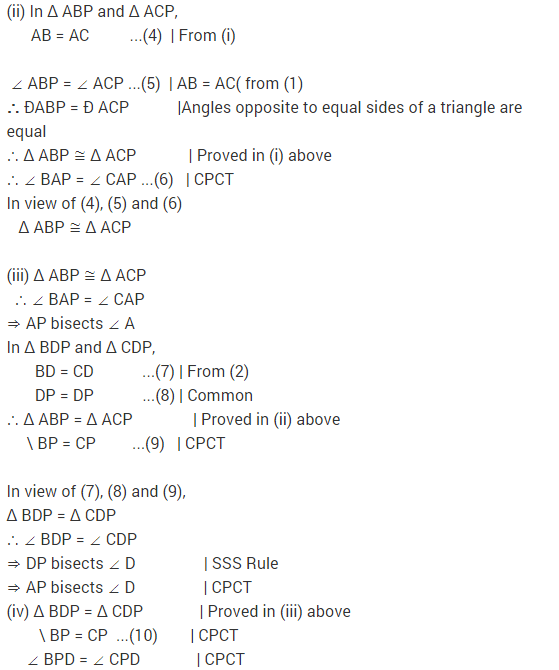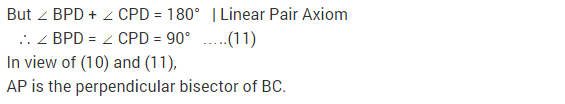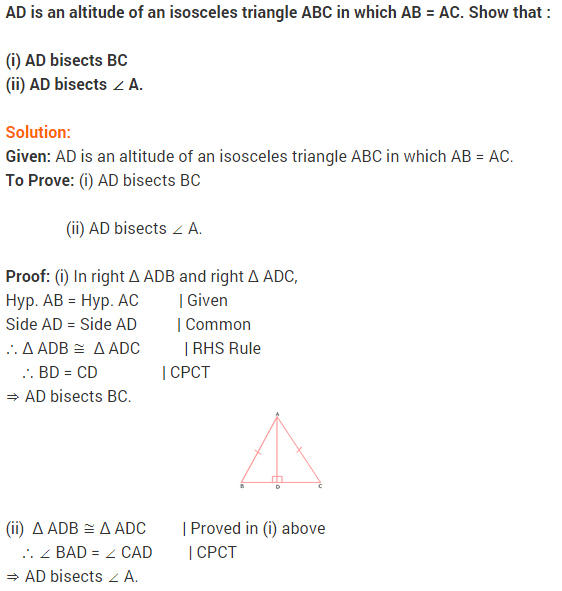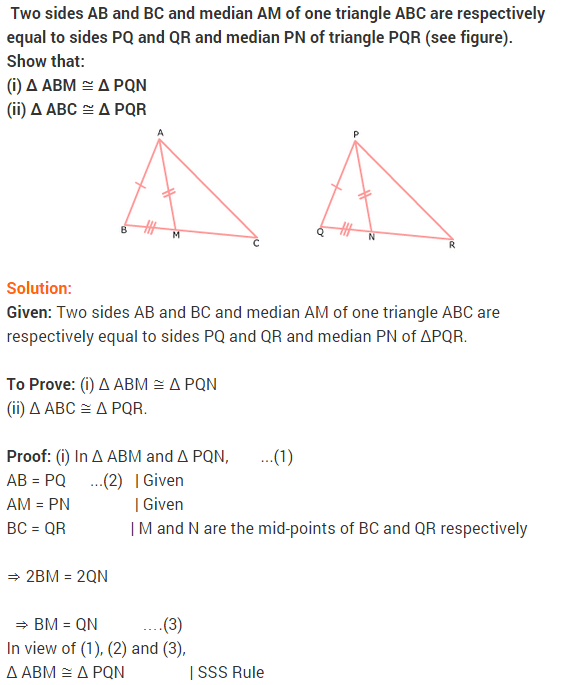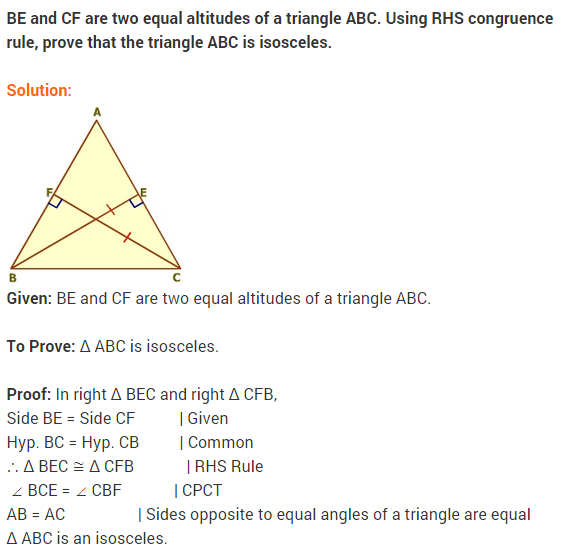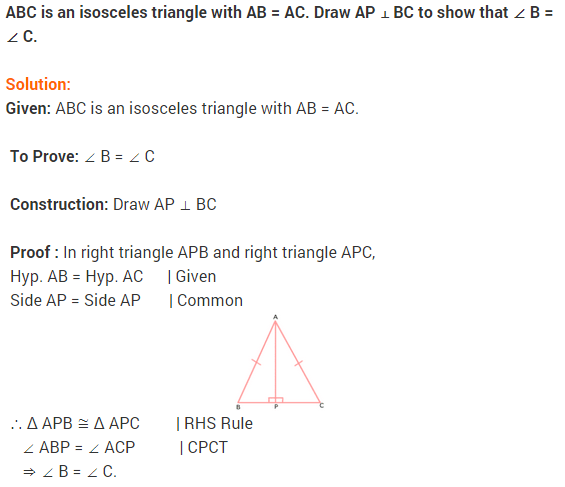### NCERT Solutions for Class 9 Maths Chapter 7 Triangles Ex 7.4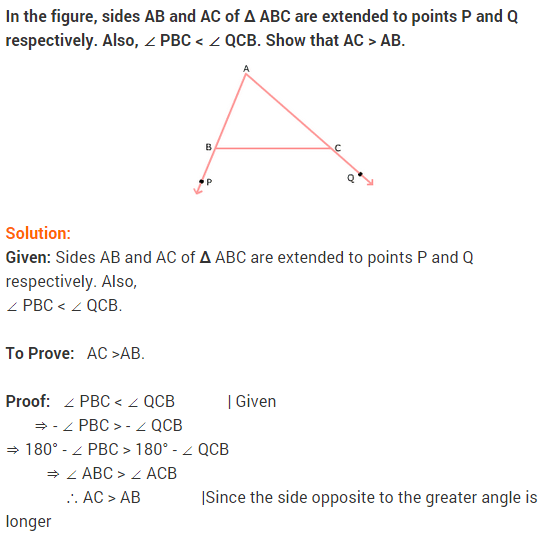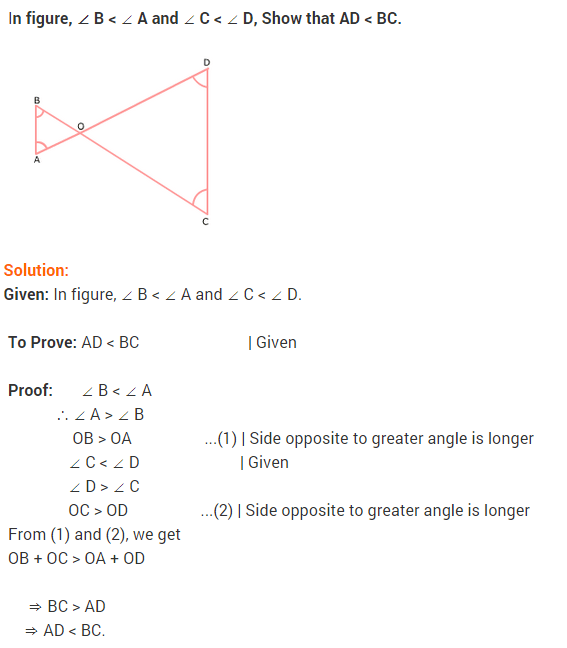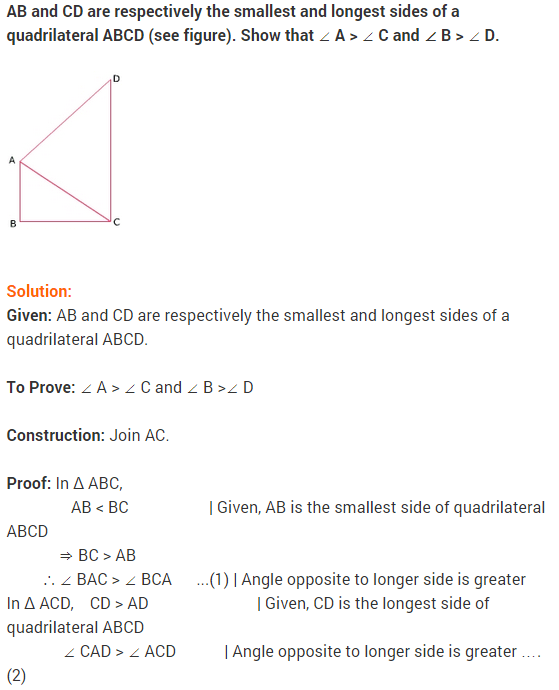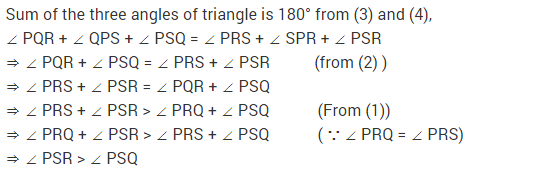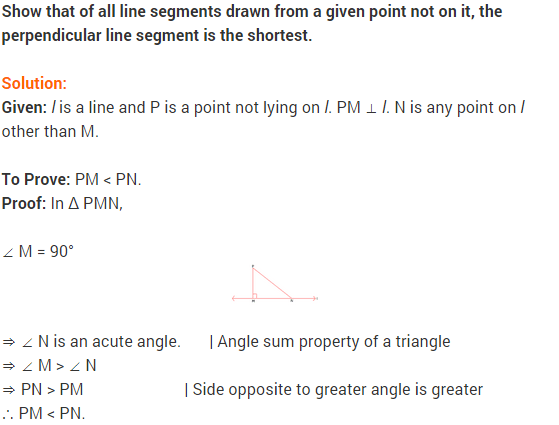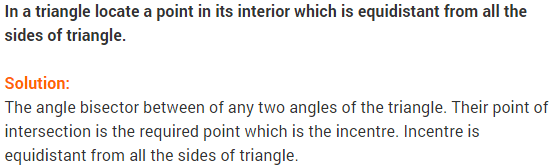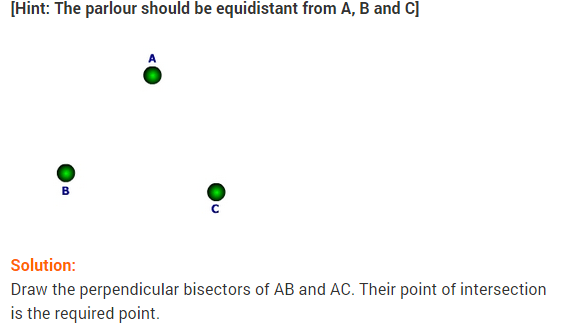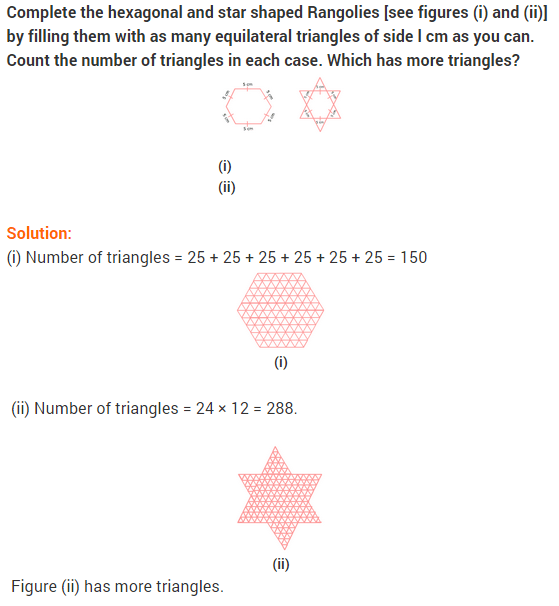### NCERT Solutions for Class 9 Maths Chapter 7 Triangles Ex 7.5

Question 1.
ABC is a triangle. Locate a point in the interior of ∆ ABC which is equidistant from all the vertices of ∆ ABC.
Solution:
Suppose OM and ON be the perpendicular bisectors of sides BC and AC of ∆ ABC.So, O is equidistant from two endpoints 0 and C of line segment BC as O lies on the perpendicular bisector of BC. Similarly, O is equidistant from C and A Hence, O be an orthocentre of ∆ABC.

Question 2.
In a triangle locate a point in its interior which is equidistant from all the sides of the triangle.
Solution:
Suppose BN and CM be the bisectors of ∠ ABC and ∠ ACB, respectively intersect AC and AB at N and M, respectively.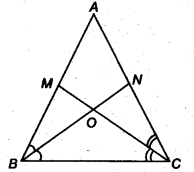Since, O lies on the bisector BN of ∠ ABC, so O will be equidistant from BA and BC. Again, O lies on the bisector CM of ∠ ACB.
So, O will be equidistant from CA and BC. Thus, O will be equidistant from AB, BC and CA Hence, O be a circumcentre of ∆ABC.

Question 3.
In a huge park, people are concentrated at three points (see figure)
A: where these are different slides and swings for children.
B: near which a man-made lake is situated.
C: which is near to a large parking and exist.
Where should an ice-cream parlor be set? up so that maximum number of persons can approach it?
[Hint The parlour should be equidistant from A, B and C.]Solution:
The ice-cream parlor should be equidistant from A B and C for which the point of intersection of perpendicular bisectors of AB, BC, and CA should be situated.
So, O is the required point which is equidistant from A B and C.

Question 4.
Complete the hexagonal and star shaped Rangolies [see Fig. (i) and (ii)] by filling them with as many equilateral triangles of side 1 cm as you can. Count the number of triangles in each case. Which has more triangles?Solution:
We first divide the hexagon into six equilateral triangles of side 5cm as follow.We take one triangle from six equilateral triangles as shown above and make as many equilateral triangles of one side 1 cm as shown in the figure.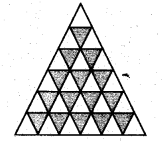The number of equilateral triangles of side 1 cm = 1 + 3 + 5 + 7 + 9 = 25
So, the total number of triangles in the hexagon = 6x 25 = 150
To find the number of triangles in the Fig. (ii), we adopt the same procedure.
So, the number of triangles in the Fig. (ii) = 12 x 25 = 30Q Hence, Fig. (ii) has more triangles.

## Triangles Class 9 Extra Questions Maths Chapter 7

Extra Questions for Class 9 Maths Chapter 7 Triangles## SabDekho

The Complete Educational Website